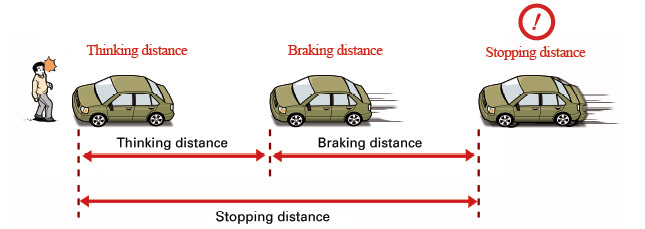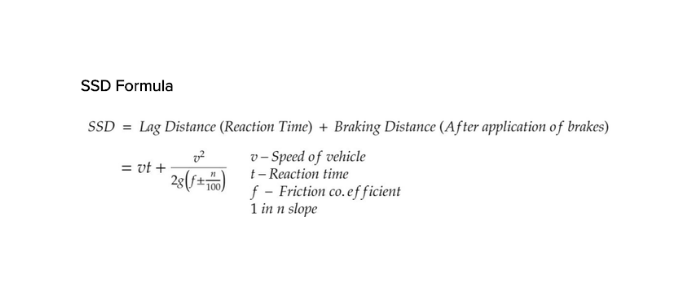• ## Stopping Sight Distance Calculation• Kinetic energy is equated to the work done to apply the brakes - Braking distance

• Reaction time varies. For young drivers it can be 1-2 sec and older drivers it can be as high as 3-4 seconds.

• SSD will be more when you are going downhill (downward slope). Use minus n/100 in formula. For upward slope, use +n/100.

• Friction coefficient varies from 0.3-0.4

LAG DISTANCE

The distance traveled by vehicle at uniform design speed during the total reaction time(Tr) is termed as lag distance.

QUESTION- Calculate the lag distance for design speed of 47 km/h for two-way traffic on a single-lane road (assume coefficient of friction as 0.38 and reaction time of driver as 2.5 seconds)

Concept: Lag distance = 0.278 × V × tR Where, V = Speed in Kmph tR = Reaction time in sec

Calculation: Lag distance = 0.278 × 47 × 2.5 = 32.665

For two way traffic on a single lane lag distance = 2 × 32.665 = 65.33

# Stopping distance = reaction distance + braking distance

• Reaction distance

The reaction distance is the distance you travel from the point of detecting a hazard until you begin braking or swerving.

### The reaction distance is affected by

• The car’s speed (proportional increase):

• 2 x higher speed = 2 x longer reaction distance.

• 5 x higher speed = 5 x longer reaction distance.

• Normally 0.5–2 seconds.

• 45–54 year-olds have the best reaction time in traffic.

• 18–24 year-olds and those over 60 have the same reaction time in traffic. Young people have sharper senses but older people have more experience.

### The reaction distance can be decreased by

• Anticipation of hazards.

• Preparedness.

### The reaction distance can be increased by

• The necessity of decision-making (for example, between braking or steering out of the way).

• Alcohol, drugs and meditation.

Easy method: Calculate the reaction distance

Formula: Remove the last digit in the speed, multiply by the reaction time and then by 3.

Example of calculation with a speed of 50 km/h and a reaction time of 1 second

50 km/h ⇒ 5

5 * 1 * 3 = 15 meters reaction distance

More precise method: Calculate the reaction distance

Formula: d = (s * r) / 3.6

d = reaction distance in meters (to be calculated).

s = speed in km/h.

r = reaction time in seconds.

3.6 = fixed figure for converting km/h to m/s.

Example of calculation with a speed of 50 km/h and a reaction time of 1 second:

(50 * 1) / 3.6 = 13.9 meters reaction distance